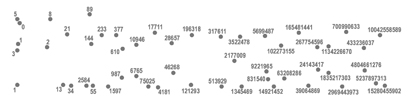DOT-TO-DOT

Graphically and conceptually, the format of the dot-to-dot allows one to enter out of nothing/zero (from the dot, to the line, to the plane) with the numbers providing a narrative for navigation and the line created demonstrating the path traversed. This process highlights the fact that a sequence of numbers is both a static arrangement and a formation that implies and allows movement. The standard 'whole number' progression of 1, 2, 3, 4, 5... that normally directs one's pencil around the constellation of a dot-to-dot is understood as moving forwards/positively in surmountable steps of one. The navigator is not asked to question the number pattern, only deliberate and then discover that which the formation of dots will depict. If one were to make a dot-to-dot and used the numbers of the Fibonacci sequence, for example, [0, 1, 1, 2, 3, 5, 8, 13, 21, 34, etc...] the task presented for the navigator alters. In this case, one is asked to understand the operating process of the sequence before one can begin to navigate the points. Once it becomes clear that the pattern is based upon the next number being the sum of the previous two [0, 1, (0+1)= 1, (1+1)= 2, (2+1)= 3, (2+3)= 5, (3+5)= 8, etc...] the movement between the dots can proceed. "The number is no longer a means of counting or measuring but of moving" (Deleuze and Guattari, 1988:389).RELATED PAGES:
For a dot-to-dot that does not use numbers as it's navigating system... see >>GREY MATTERS
Two dots provide a line, three dots enable a triangle... see >>THE TRIANGLE PROBLEM

WHAT

ZOE LAUGHLIN

HOME